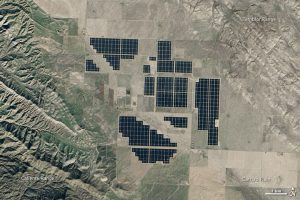Solar energy for everyone, this is a dream. But how many solar panels would be required to cover such energy need?
To provide energy through solar panels, would be sufficient to cover the 2% of the surface of the Sahara desert (it is equivalent to the size of the Portugal): solar radiation in North Africa is actually three times higher than the average European. To meet European needs, it would be enough to cover 0.3% of the surface of this desert. A relatively small proportion, considering that in the world are consumed each year more than 20,000 terawatt-hour of electricity (a million million watt-hours), including approximately 3200 in Europe (see figures).

In a square shape, this would be about 570 kilometres on a side.The Topaz solar farm seen from space. With a photovoltaic surface of 25 km2 the set is capable of producing 1100 GWh (gigawatt-hour) per year. It's the second most powerful solar power plant in the world (after Solar Star, SunPower). Earth Observatory image, 2015.

There are about 7.5 billion human beings on the planet.

Approx 9 square metres of solar panels will generate 1 kW energy for about 6 hours a day.

So the total panel area needed, in square kilometres, is calculated as follows:

Total energy needed per day

= E = (20 x 60 x 24 x 7.5 x 10^9) watthours

1 square kilometre of panels generate energy

= D = 6 x 10^6 x 10^3 / 9 watthours per day.

Number of square kilometres needed

= E/D = 324000

A few issues...
To begin with, very high temperatures and desert sand storms would decrease the efficiency of the panels. However, it would be possible to use special protection coverages, which slow down the deterioration and improve energy efficiency at high temperature. Another problem would be the transmission of electrical energy in a way effective over very long distances. A solution could come from the HVDC (High Voltage Direct Current). It is a technology of high-voltage energy transport and running continuous (usually, the transport is done running alternative), which allows a drastic reduction in losses and therefore an increase in the overall performance of the system.